# Second Law of Thermodynamics Second Law of Thermodynamics

• Slides: 14Second Law of ThermodynamicsSecond Law of Thermodynamics No cyclic process that converts heat entirely into work is possible. ¡ W can never be equal to Q. ¡ Some energy must always be transferred as heat to the system’s surrounding. ¡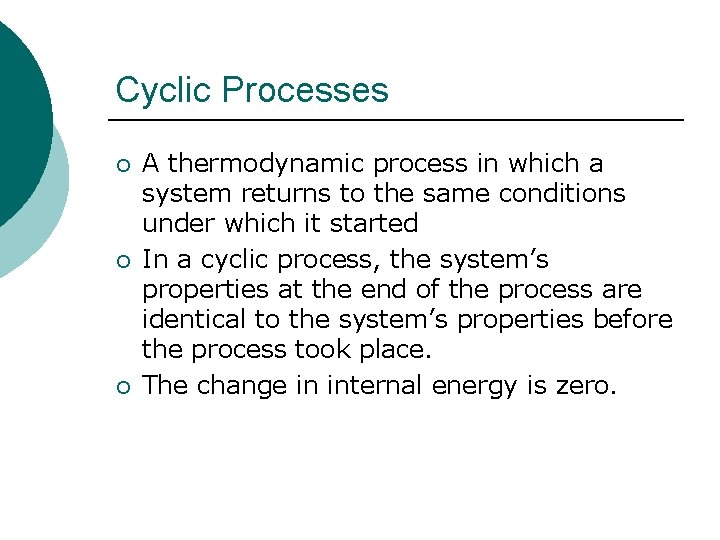Cyclic Processes ¡ ¡ ¡ A thermodynamic process in which a system returns to the same conditions under which it started In a cyclic process, the system’s properties at the end of the process are identical to the system’s properties before the process took place. The change in internal energy is zero.Efficiency is a measure of how well an engine operates. ¡ Efficiency=Wnet = Qh-Qc = 1 - Qh ¡ Qh Qh Qc Qh = energy removed as heat ¡ Qc= energy added as heat ¡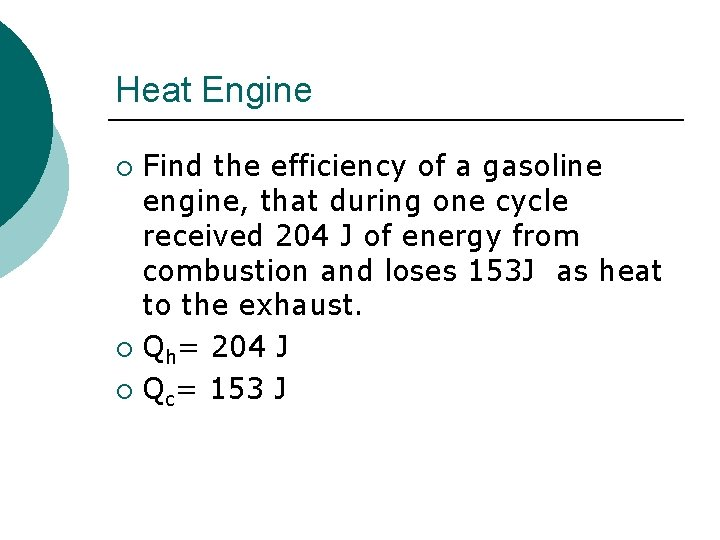Heat Engine Find the efficiency of a gasoline engine, that during one cycle received 204 J of energy from combustion and loses 153 J as heat to the exhaust. ¡ Qh= 204 J ¡ Qc= 153 J ¡Heat Engine Choose an equation ¡ 1 - Qc/Qh ¡ 1 -153/204 ¡ =. 250 ¡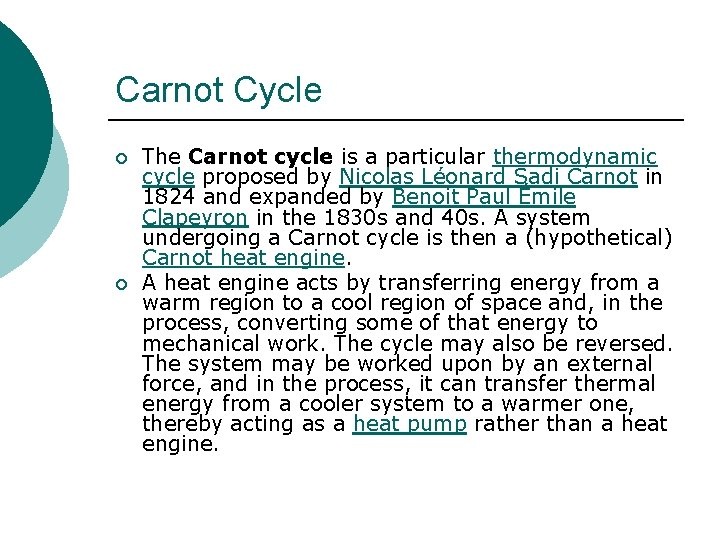Carnot Cycle ¡ ¡ The Carnot cycle is a particular thermodynamic cycle proposed by Nicolas Léonard Sadi Carnot in 1824 and expanded by Benoit Paul Émile Clapeyron in the 1830 s and 40 s. A system undergoing a Carnot cycle is then a (hypothetical) Carnot heat engine. A heat engine acts by transferring energy from a warm region to a cool region of space and, in the process, converting some of that energy to mechanical work. The cycle may also be reversed. The system may be worked upon by an external force, and in the process, it can transfer thermal energy from a cooler system to a warmer one, thereby acting as a heat pump rather than a heat engine.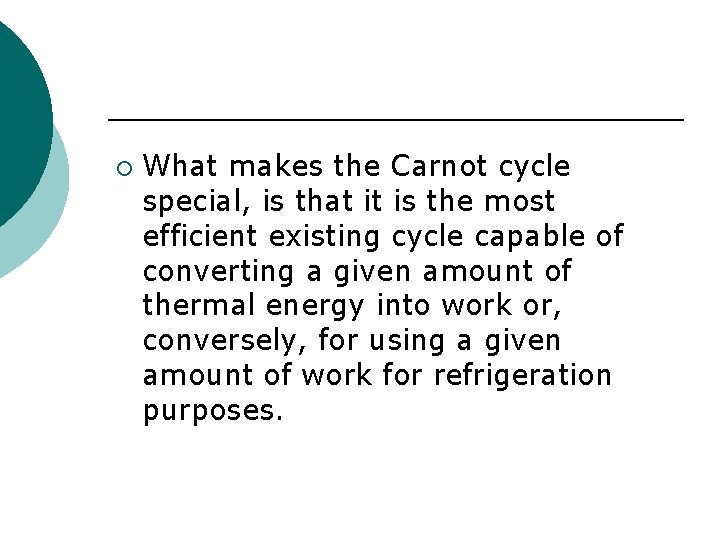¡ What makes the Carnot cycle special, is that it is the most efficient existing cycle capable of converting a given amount of thermal energy into work or, conversely, for using a given amount of work for refrigeration purposes.The Carnot cycle when acting as a heat engine consists of the following steps: ¡ 1. Reversible isothermal expansion of the gas at the "hot" temperature, TH (isothermal heat addition). During this step (A to B on Figure 1, 1 to 2 in Figure 2) the expanding gas makes the piston work on the surroundings. The gas expansion is propelled by absorption of quantity Q 1 of heat from the high temperature reservoir.¡ 2. Isentropic (Reversible adiabatic) expansion of the gas (isentropic work output). For this step (B to C on Figure 1, 2 to 3 in Figure 2) the piston and cylinder are assumed to be thermally insulated, thus they neither gain nor lose heat. The gas continues to expand, working on the surroundings. The gas expansion causes it to cool to the "cold" temperature, TC¡ 3. Reversible isothermal compression of the gas at the "cold" temperature, TC. (isothermal heat rejection) (C to D on Figure 1, 3 to 4 on Figure 2) Now the surroundings do work on the gas, causing quantity Q 2 of heat to flow out of the gas to the low temperature reservoir.¡ 4. Isentropic compression of the gas(isentropic work input). (D to A on Figure 1, 4 to 1 in Figure 2) Once again the piston and cylinder are assumed to be thermally insulated. During this step, the surroundings do work on the gas, compressing it and causing the temperature to rise to TH. At this point the gas is in the same state as at the start of step 1.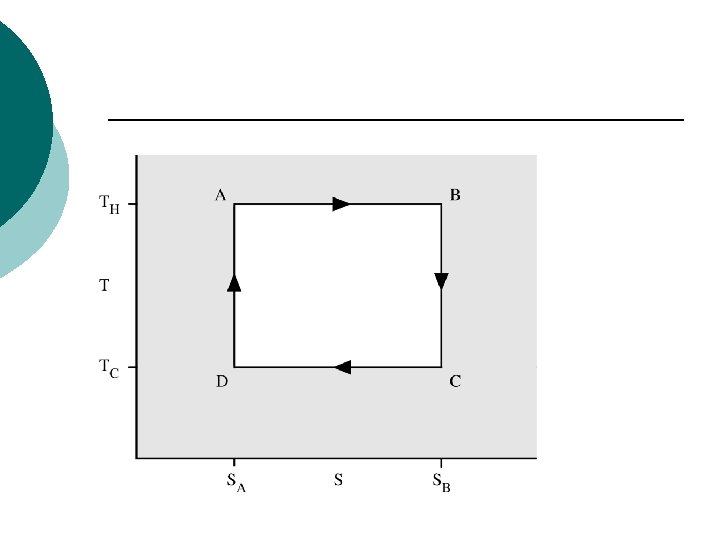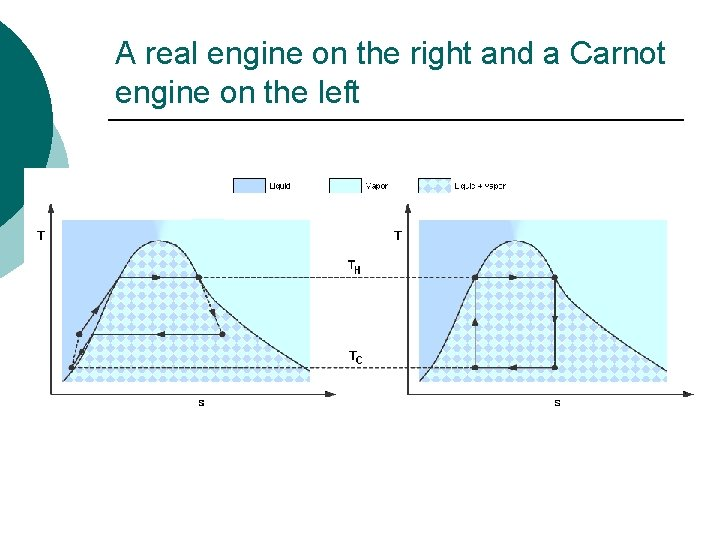A real engine on the right and a Carnot engine on the left